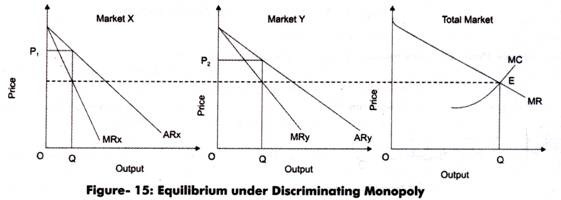# Price and Output Decisions in Discriminating Monopoly

Price discrimination is a strategy used by a monopolist under which a given product is sold at different prices.

The main aim of a monopolist of practicing price discrimination strategy is to increase total revenue and earn maximum profit.

However, in case of discriminating monopoly, different prices are charged for output. Let us now discuss how a monopolist decides the equilibrium price and output under the discriminating monopoly with the help of an example.

Assume that a monopolist, Mr. X has divided market into two submarkets, X and Y. He found that the elasticity of demand is greater in market Y than in market X.

The monopolist would earn maximum profits where MR=MC and MC curve cuts MR curve from below, which is shown in Figure-15:In Figure-15, in case of total market, output OQ has to be distributed in such a way that MR equals MC at E. Thus, a monopolist will sell output OQ1 in market X and OQ2 in market Y as at these levels of output equals QE that is an equilibrium output. The price charged in both the markets will be equal to AR or demand curve.